﻿ 基于系统动力学模型的物元相关网研究
«上一篇文章快速检索 高级检索

 智能系统学报2017, Vol. 12Issue (4): 459-467  DOI: 10.11992/tis.2016100130

### 引用本文LI Wenjun, YANG Chunyan. Research on a correlative network of matter-elements based on a system dynamics model[J]. CAAI Transactions on Intelligent Systems, 2017, 12(4): 459-467. DOI: 10.11992/tis.201610013.### 文章历史

1. 广东工业大学 可拓学与创新方法研究所, 广东 广州 510006;
2. 广东工业大学 机电工程学院, 广东 广州 510006

Research on a correlative network of matter-elements based on a system dynamics model
LI Wenjun1,2, YANG Chunyan1,21. Research Institute of Extenics and Innovation Methods, Guangdong University of Technology, Guangzhou 510006, China;
2. School of Electromechanical Engineering, Guangdong University of Technology, Guangzhou 510006, China
Abstract: In the correlative analysis of Extenics, the key is to define the correlation function relating to the basic-elements. However, it is very difficult to define the correlation function when it is applied to specific areas and this limits the usage of the correlative network. In this paper, a correlation network based on matter-elements is studied. Then, we present a method for establishing a system dynamics model of the correlation network and a method for approximately identifying the correlation function based on the system dynamics model, in which the correlation functions under some related matter-elements are unknown. The principle of this method is to derive the approximate correlation functions of related matter-elements according to the principle of collecting and analyzing basic data, and establishing a kinetic model for simulation and analysis in terms of a fixed procedure in the correlative network. In this way, correlative analysis becomes easy and can be simulated. The clear conclusion is that the method efficiently simulates the correlation function and lays the foundation for the application of correlation analysis to specific areas.
Key words: Extenics    matter-element    correlative analysis    correlative network    system dynamics    simulation    correlation functions    modeling

1 相关分析与系统动力学建模 1.1 相关分析理论

1.2 相关网方法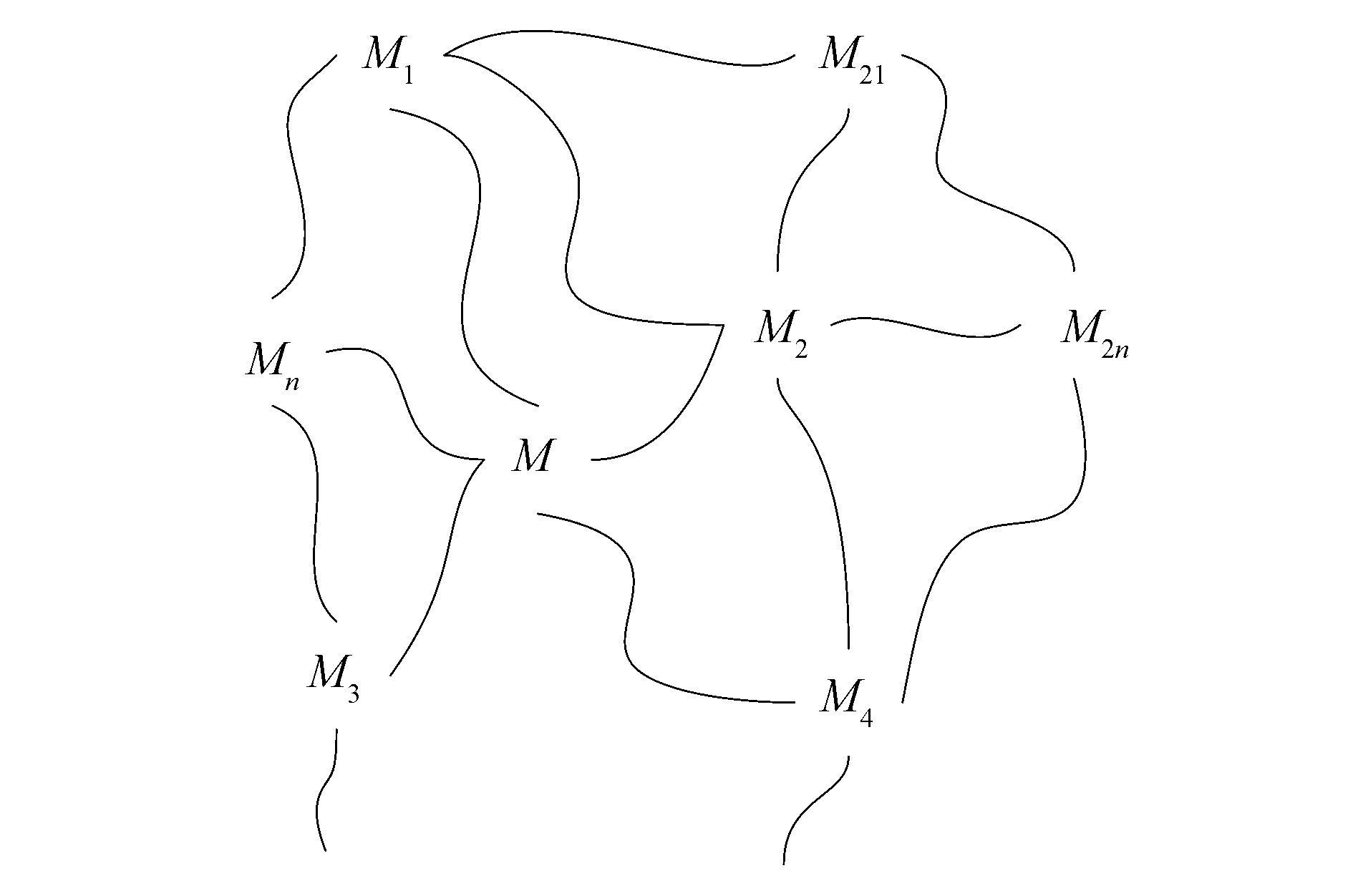图 1 相关网符号表示 Fig.1 Symbolic representation of correlated network

1) 将要分析的事物，建立初始物元模型Mi(t)；

2) 利用相关分析原理和专业知识列出物元Mi(t)的相关网；

3) 对相关网进行分析，确定引起物元Mi(t)变化的物元Mj(t)，或由于物元Mi(t)的变化而引起变化的物元Mj(t)。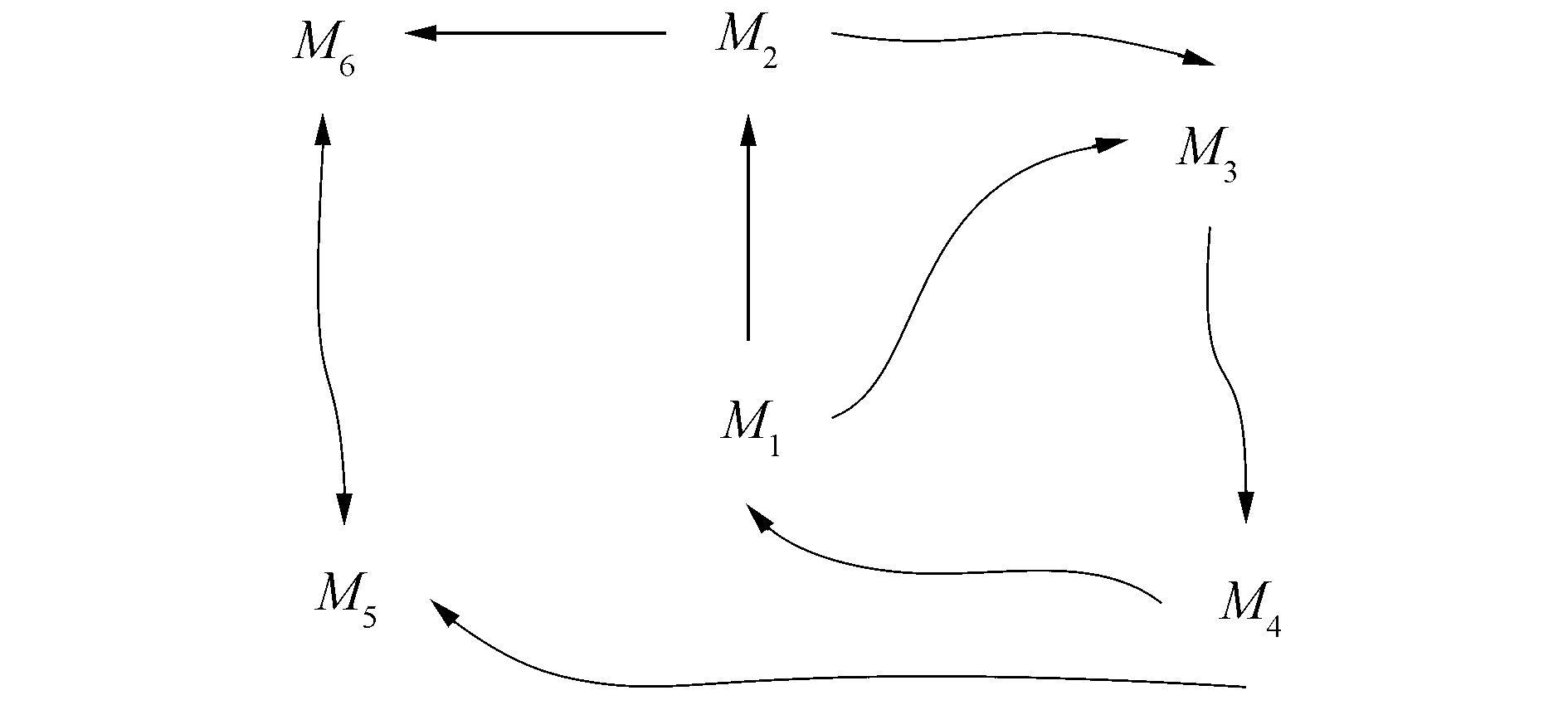图 2 6个物元形成的相关网 Fig.2 The correlative network between six matter-elements
1.3 系统动力学模型的建模流程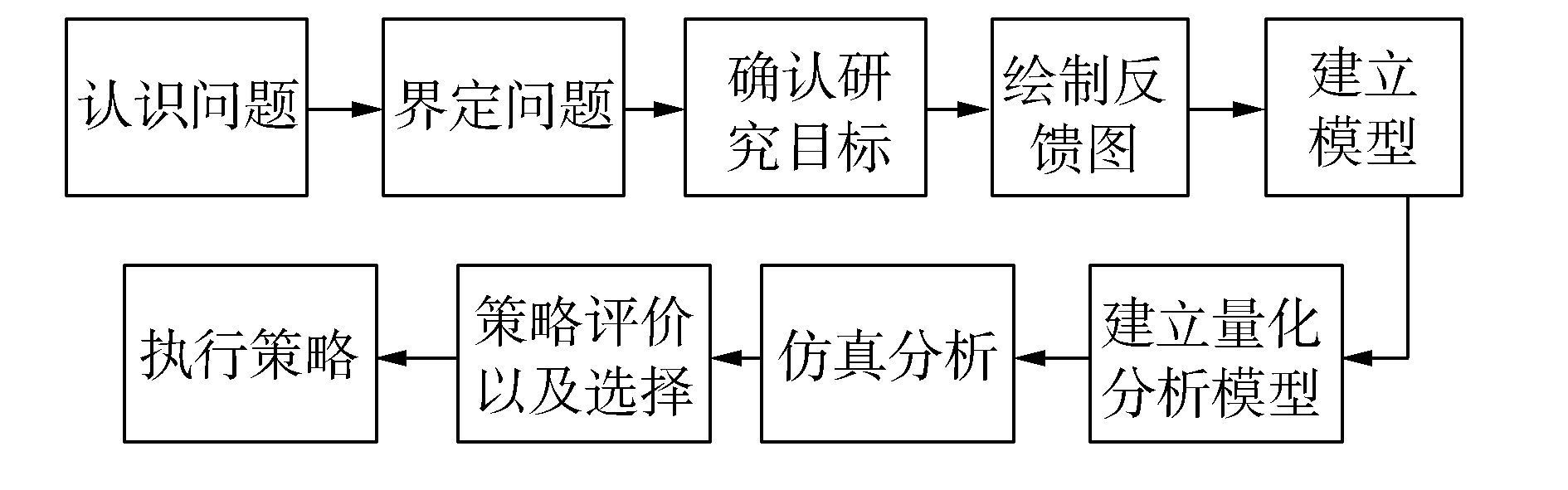图 3 建模流程图 Fig.3 Flow of modeling process

2 基于系统动力学模型的相关网建模方法

1) 针对具体研究对象的建立原始物元模型Mi(t)=(O (t), C, V(t))，建立的物元模型的维数和个数依具体研究对象而定，可以是若干个物元模型，其中的模型可以是一维模型，也可以是多维模型。

2) 根据建立的物元模型选取相关的同物物元和异物物元，建立相关网，然后分析各物元的量值之间的函数关系。

3) 建立物元相关网图。相关网是在相关的物元中表示有关系的符号连起来所组成的网状图形。但是相关网中并不能表示一个线段的两个端点物元的特征cicj的量值之间具体的关系：函数关系、模糊函数关系以及cicj两者的量值之间谁是自变量，谁是因变量等关系。在明确所有相关物元的量值之间的函数关系的情况下，才能做进一步的模拟分析。

4) 以包含特征ci的一维物元为研究对象，利用系统动力学软件Venple对相关网图进行变形，称其为“动力学相关网”。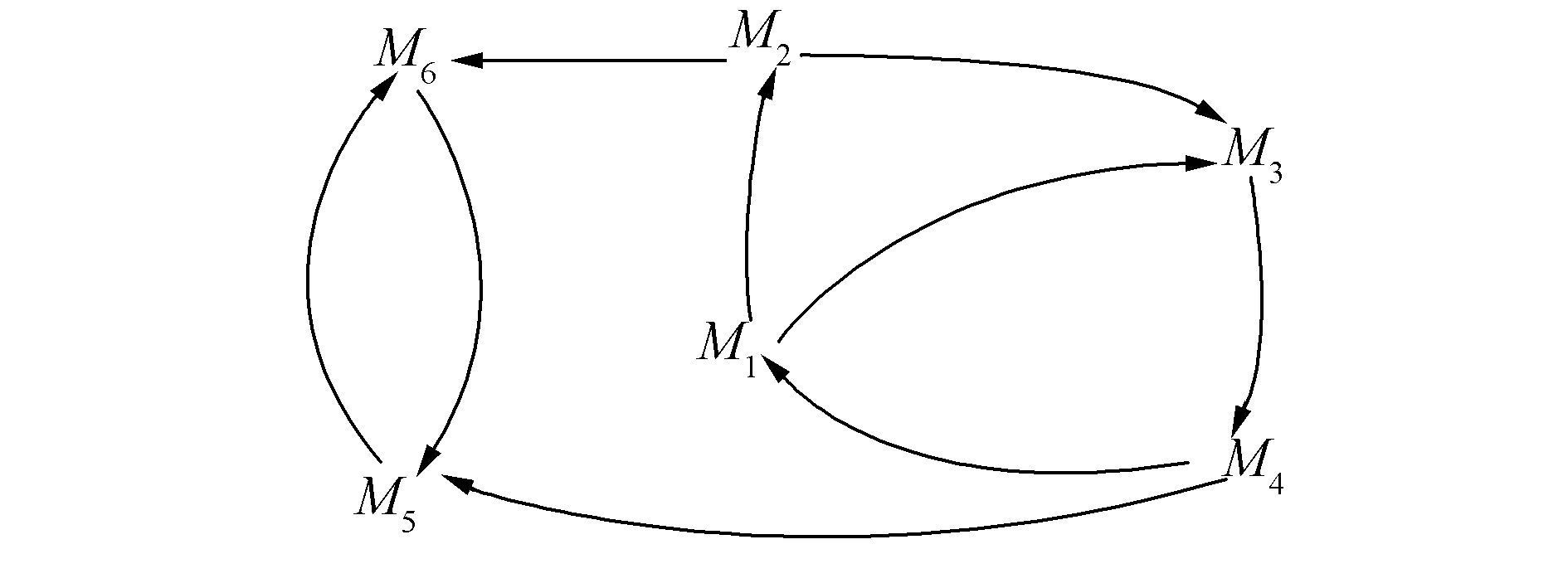图 4 动力学相关关系网 Fig.4 The correlation network of dynamics

5) 结合特征之间的关联传递关系，建立动力学模型，并进行模拟分析。

① 根据有向线段两端的物元的特征cicj之间的正影响和负影响关系，在线段的箭头处用符号“+”和“－”标出，调整相关回路，并将回路用符号表示，如图 5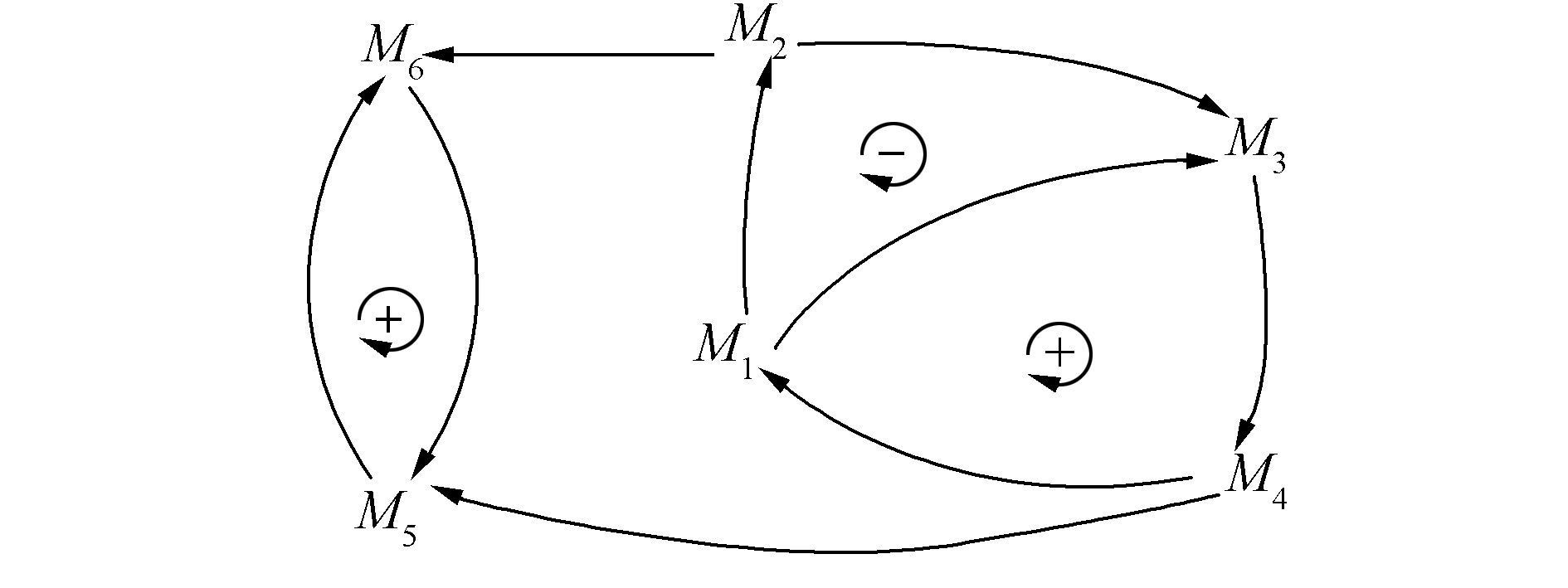图 5 动力学相关回路图 Fig.5 The correlation loop of dynamics

② 建立相关分析目标的存量流量图。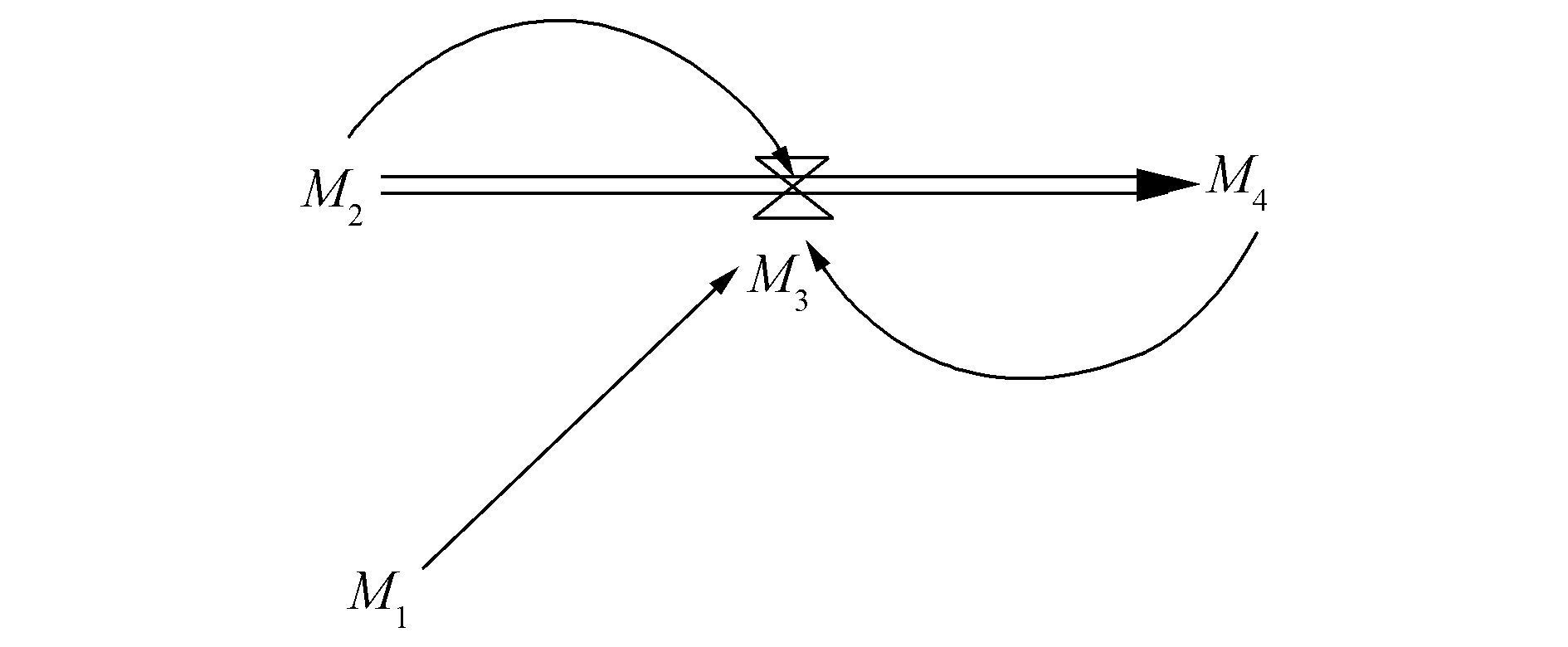图 6 M4的存量流量图 Fig.6 The stock and flow diagram of M4

③ 量化分析模型及仿真计算。

6) 初步得出模拟结果，用于后续的分析。

3 利用SD模型的DYNAMO基本方程近似构造相关函数 3.1 SD模型的DYNAMO的基本方程

SD的主要过程之一是通过确定对象系统的水准变量、速率变量、参量、辅助变量等，分析各变量之间存在的函数关系，建立DYNAMO仿真模型，进行人工计算或者仿真。这是得到描述系统内部反馈机制的流程图后建立动力学模型并进行定量分析的主要工作。DYNAMO方程就是SD的数学模型或者量化分析模型。

DYNAMO主要采用差分方程式描述有反馈的社会系统的宏观动态行为，并通过差分及代数方程式的求解(简单迭代)进行计算机仿真的专用语言。其最大特点是简单明了，容易使用。

SD的对象系统是随时间变化的动态系统。在DYNAMO方程中，变量一般都有时间标号，规定如图 7。SD使用逐步仿真的方法，仿真的时间步长记为DT。图 7 DYNAMO方程时间标号及其含义 Fig.7 Time label of DYNAMO equation and it's meaning

DYNAMO方程是SD的数学模型或者量化分析模型。基本的DYNAMO方程主要有以下3个

1) 水准方程。它是计算水准变量的方程，也就是计算一维物元特征量值的方程。它的标准形式为

 ${\rm{LEVE}}{{\rm{L}}_{{t_2}}} = {\rm{LEVE}}{{\rm{L}}_{{t_1}}} + {\rm{DT}} \cdot \left( {{\rm{RI}}{{\rm{N}}_{{t_1}{t_2}}} - {\rm{ROU}}{{\rm{T}}_{{t_1}{t_2}}}} \right)$ (1)

2) 速率方程。它是计算速率变化量的方程，用来计算从t1t2时间段内一维物元特征量值变化速率的量值。

 ${\rm{RATE}} \cdot {t_2}L = f\left( {L \cdot {t_2},A \cdot {t_2},C, \cdots } \right)$ (2)

3) 辅助方程。它是用来辅助说明速率变量的方程。

 ${\rm{AUX}} \cdot {t_2} = g\left( {A \cdot {t_2},L \cdot {t_2},{R_{{t_1}{t_2}}},C, \cdots } \right)$ (3)

3.2 近似构造相关函数的操作步骤

1) 近似构造某研究对象的相关关系时，首先要根据物元的构造规范，正确建立研究对象关于其特征和相应的量值构成的多个一维物元。确定相关的一维物元。

2) 根据领域知识，搜集研究对象关于其特征的量值变化的一些数据，需要注意这些数据的变化规律。不同的函数曲线代表着不同的函数类型。作为佐证确定的相关函数是否符合事实并且可用的依据。

3) 根据SD的基本函数模型，在符合相关数学规则的情况下，尽量从最简单的函数方程出发来近似构造特征的相关函数。如果上一次确定相关函数没有成功的话，根据上一次函数方程模拟得到的信息作为依据，逐渐将方程复杂化，继续模拟分析，直到找到可以描述事实规律的近似相关函数。

4) 利用已经建好的相关网动力学模型，在参数相同的情况下，对近似构造的函数进行建模仿真，根据搜集的数据，判断函数的正确性，如果不正确，重复上述操作，直到找到符合实际规律的近似相关函数。

4 案例分析

W公司是一家数字教学器材公司。VAX系列微型计算机是这家公司很成功的产品。每台VAX微型计算机的售价在100 000~150 000美元之间。VAX11/750型微型计算机于1980年试制成功。其销售主要是靠用户的口头传播。通过对一定数量的数据样本进行分析可知：销售曲线成正态分布，产品累计销售曲线呈现为S型结构。

4.1 建模与相关分析

1) 根据案例背景以及相关资料，建立产品的物元模型如下：

 $\begin{array}{l} M\left( t \right) = \left[ {\begin{array}{*{20}{l}} {{\rm{产品}}{O_1}\left( t \right),}&{{\rm{目标用户群总数}}{c_1},}&{{v_1}\left( t \right)}\\ {}&{{\rm{当前用户}}{c_2},}&{{v_2}\left( t \right)}\\ {}&{{\rm{潜在用户}}{c_3},}&{{v_3}\left( t \right)}\\ {}&{{\rm{销售速率}}{c_4},}&{{v_4}\left( t \right)}\\ {}&{{\rm{用户接触速率}}{c_5},}&{{v_5}\left( t \right)} \end{array}} \right] \buildrel \Delta \over = \\ \left[ {\begin{array}{*{20}{l}} {{O_1}\left( t \right),}&{{c_1},}&{{v_1}\left( t \right)}\\ {}&{{c_2},}&{{v_2}\left( t \right)}\\ {}&{{c_3},}&{{v_3}\left( t \right)}\\ {}&{{c_4},}&{{v_4}\left( t \right)}\\ {}&{{c_5},}&{{v_5}\left( t \right)} \end{array}} \right] = \left[ {\begin{array}{*{20}{l}} {{M_1}\left( t \right)}\\ {{M_2}\left( t \right)}\\ {{M_3}\left( t \right)}\\ {{M_4}\left( t \right)}\\ {{M_5}\left( t \right)} \end{array}} \right] \end{array}$

2) 根据领域知识和相关分析原理分析各分物元之间的相关关系如下：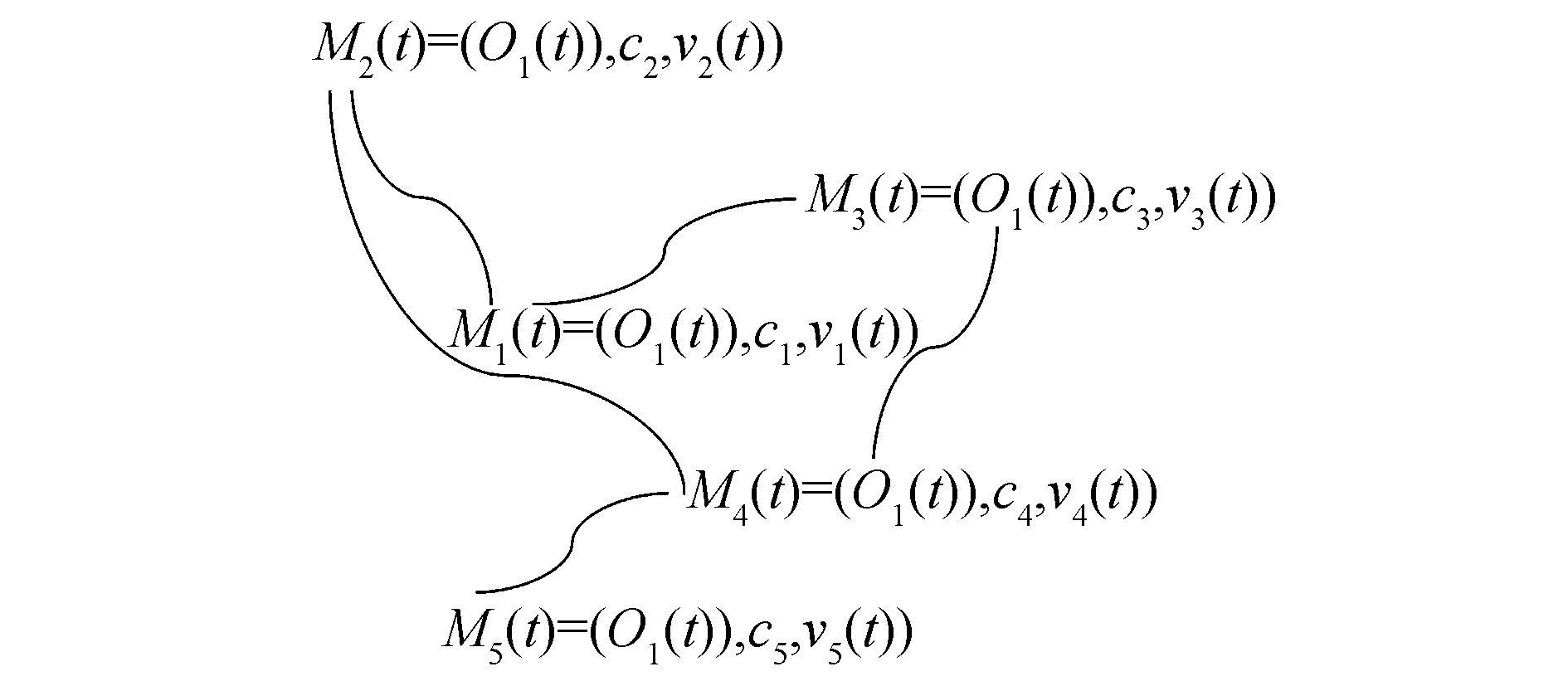图 8 相关网 Fig.8 The correlation network

3) 建立相关关系图如图 9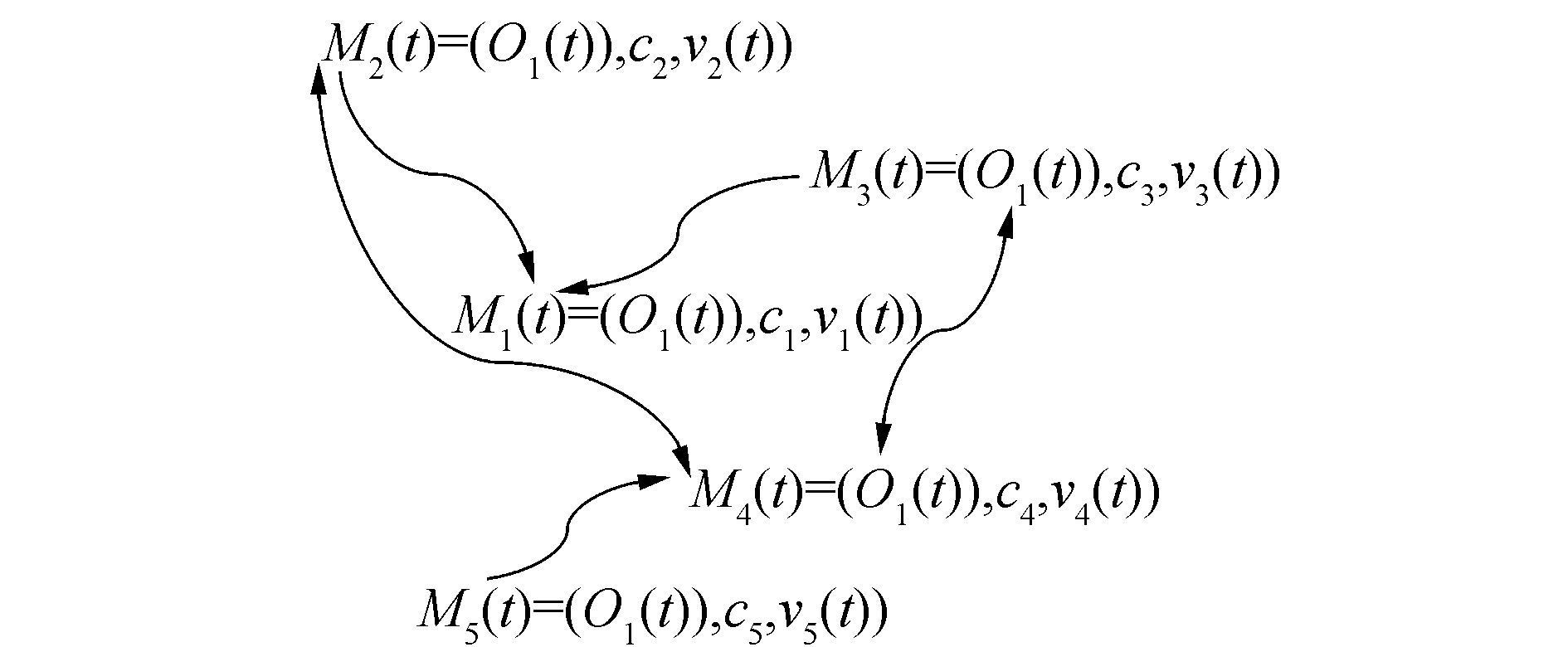图 9 相关关系图 Fig.9 The correlations of matter-elements

4) 对相关网变形得到系统动力学相关网。并分析各相关物元的量值之间的函数关系。系统动力学相关网如图 10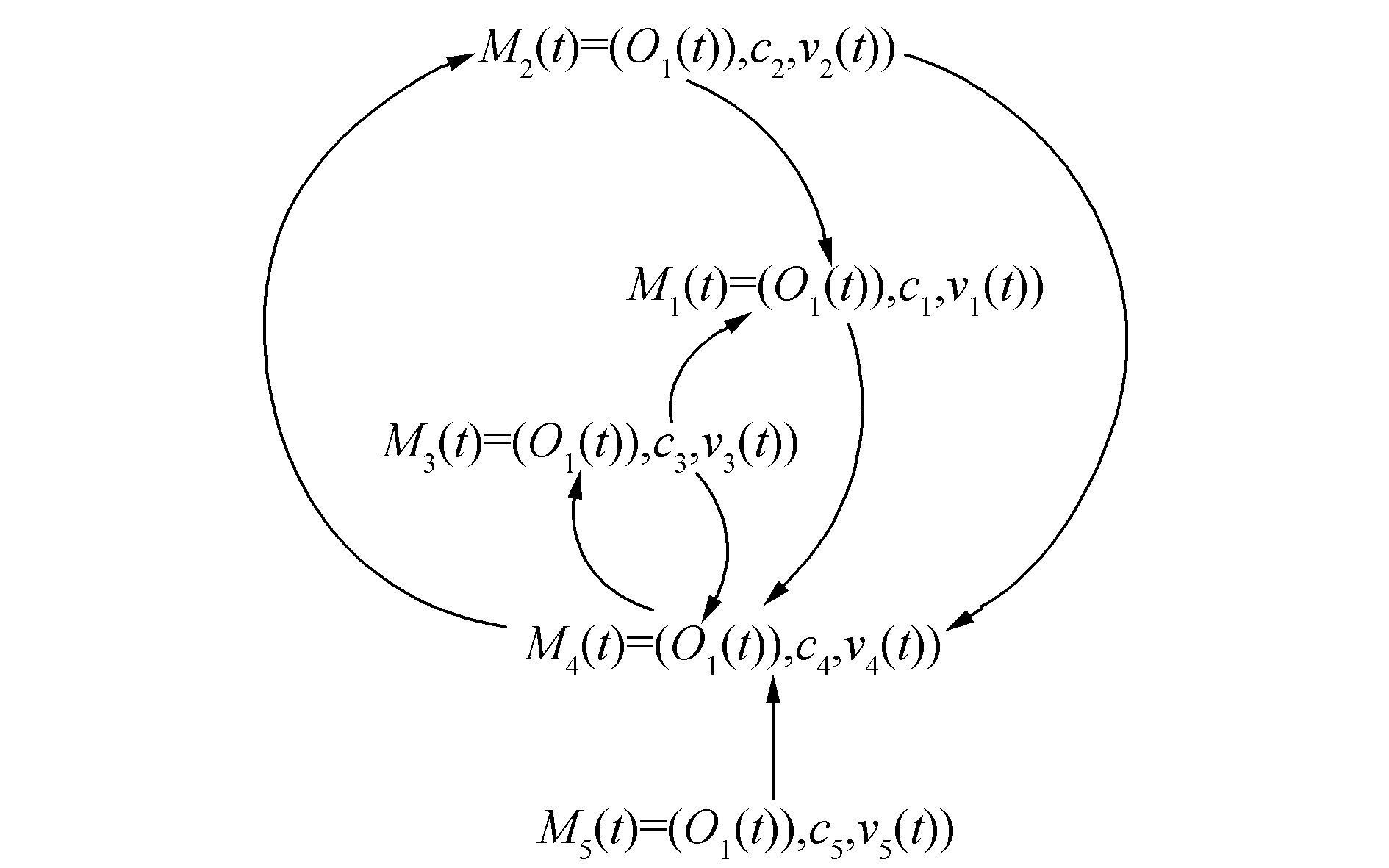图 10 系统动力学相关网 Fig.10 The correlation network of dynamics

5) 结合特征之间的关联传递关系，建立动力学模型，并进行模拟分析。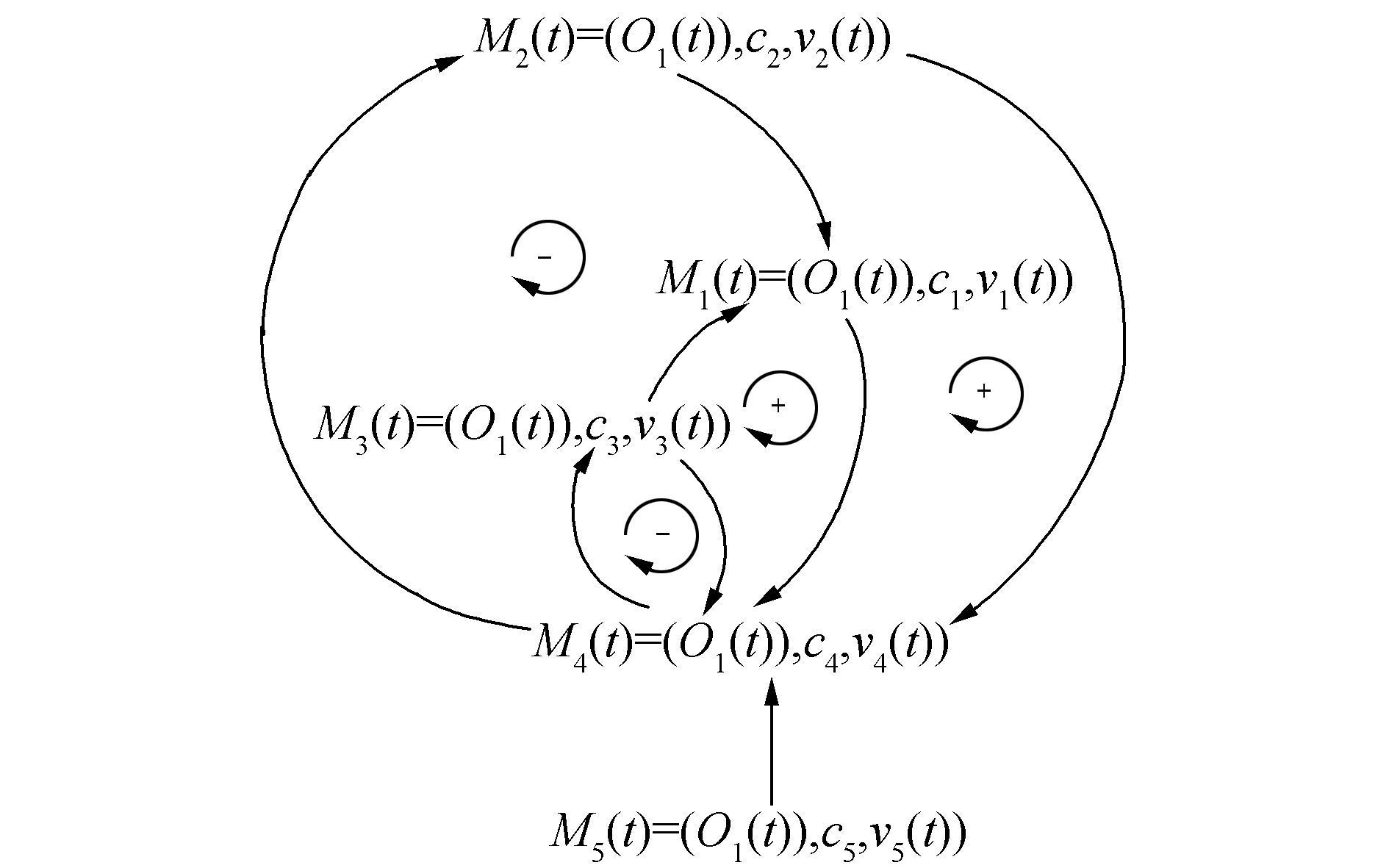图 11 动力学相关网回路图 Fig.11 The correlation loop of dynamics

6) 根据相关回路图，找出回路中存在的反馈环(回路)，并且以系统的目标为重点，提取主要回路进行分析。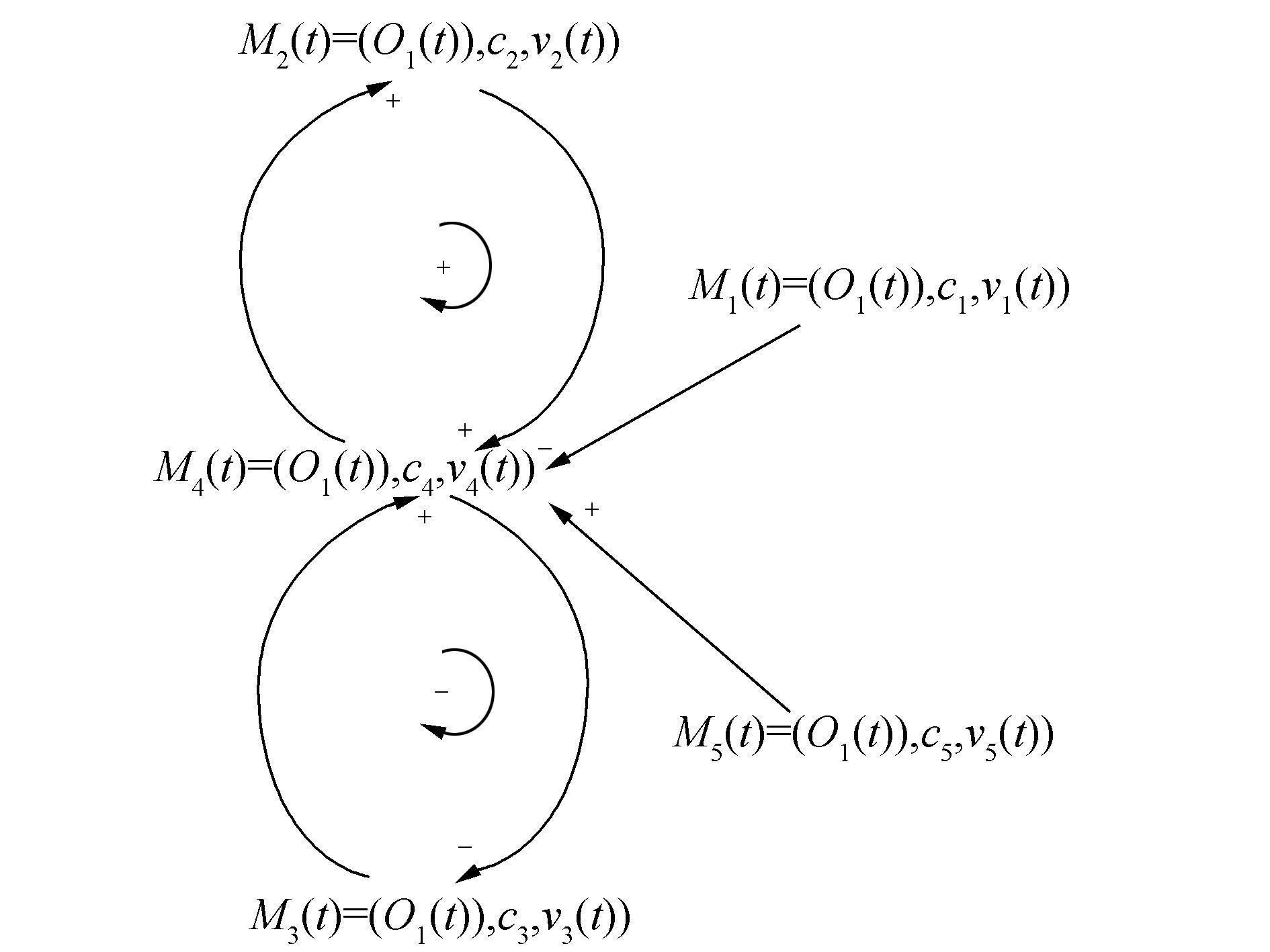图 12 销售系统回路图 Fig.12 Flow of sales system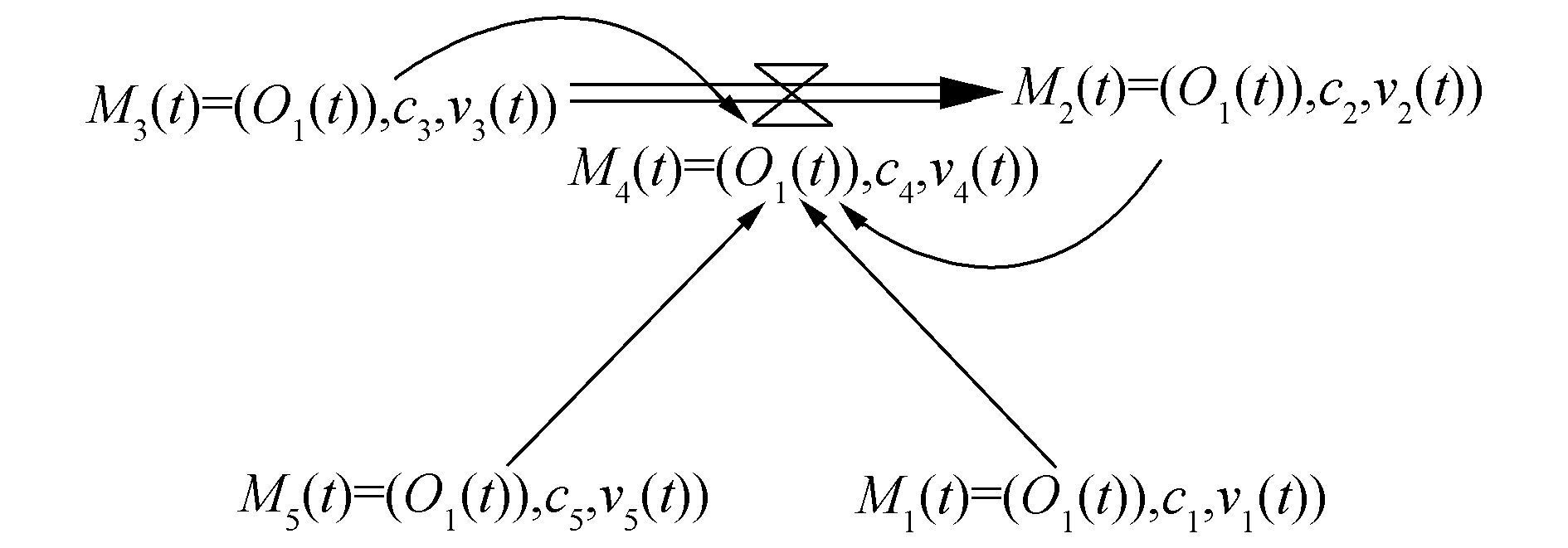图 13 销售过程存量流量图 Fig.13 The stock and flow diagram of selling progress
4.2 根据历史资料近似构造各相关函数

1) 对于不易确定的相关特征之间的相关函数，查阅并分析公司的历年的相关数据，部分如图 1415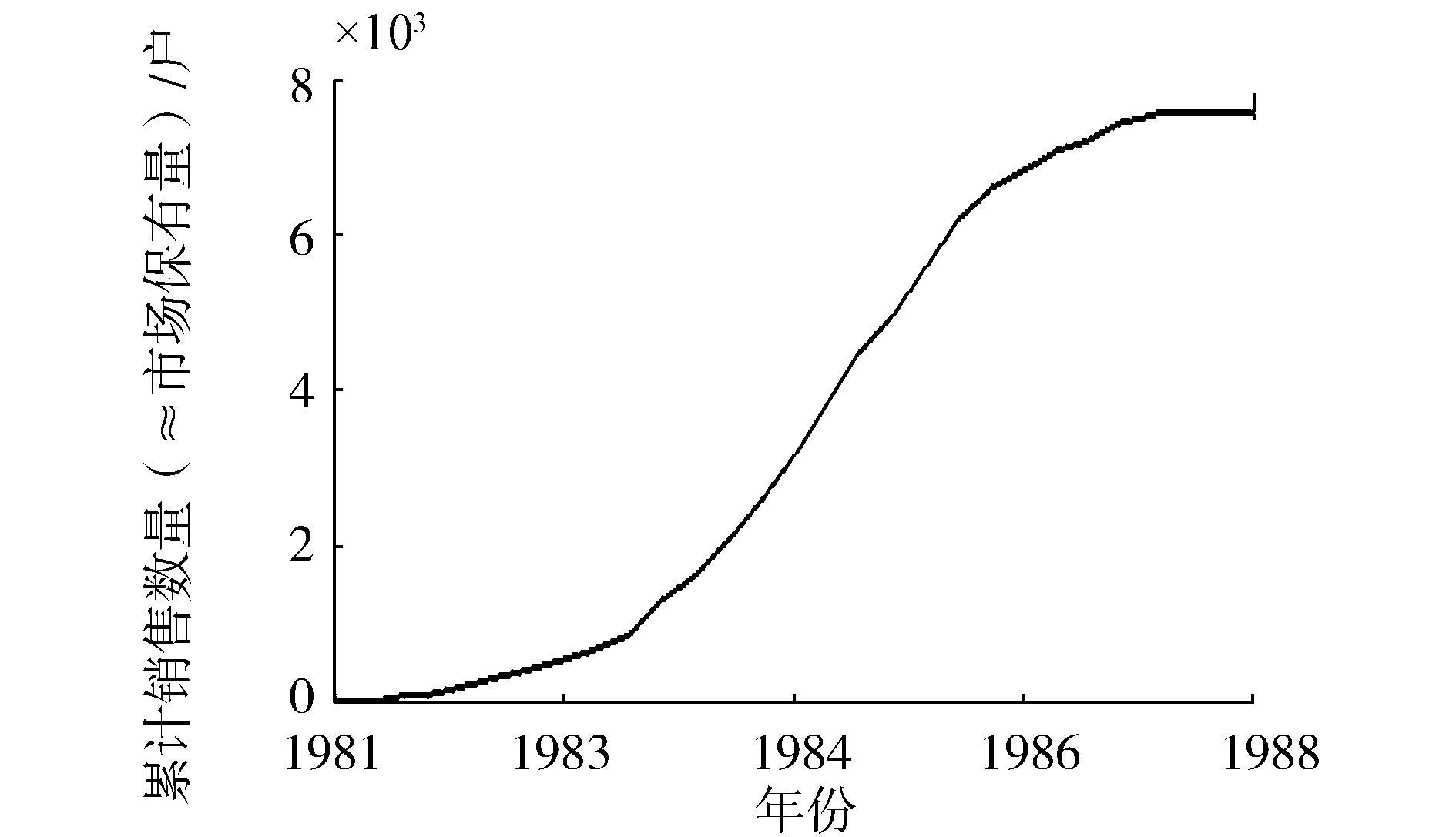图 14 累计销售量增长图 Fig.14 The growth label of total sales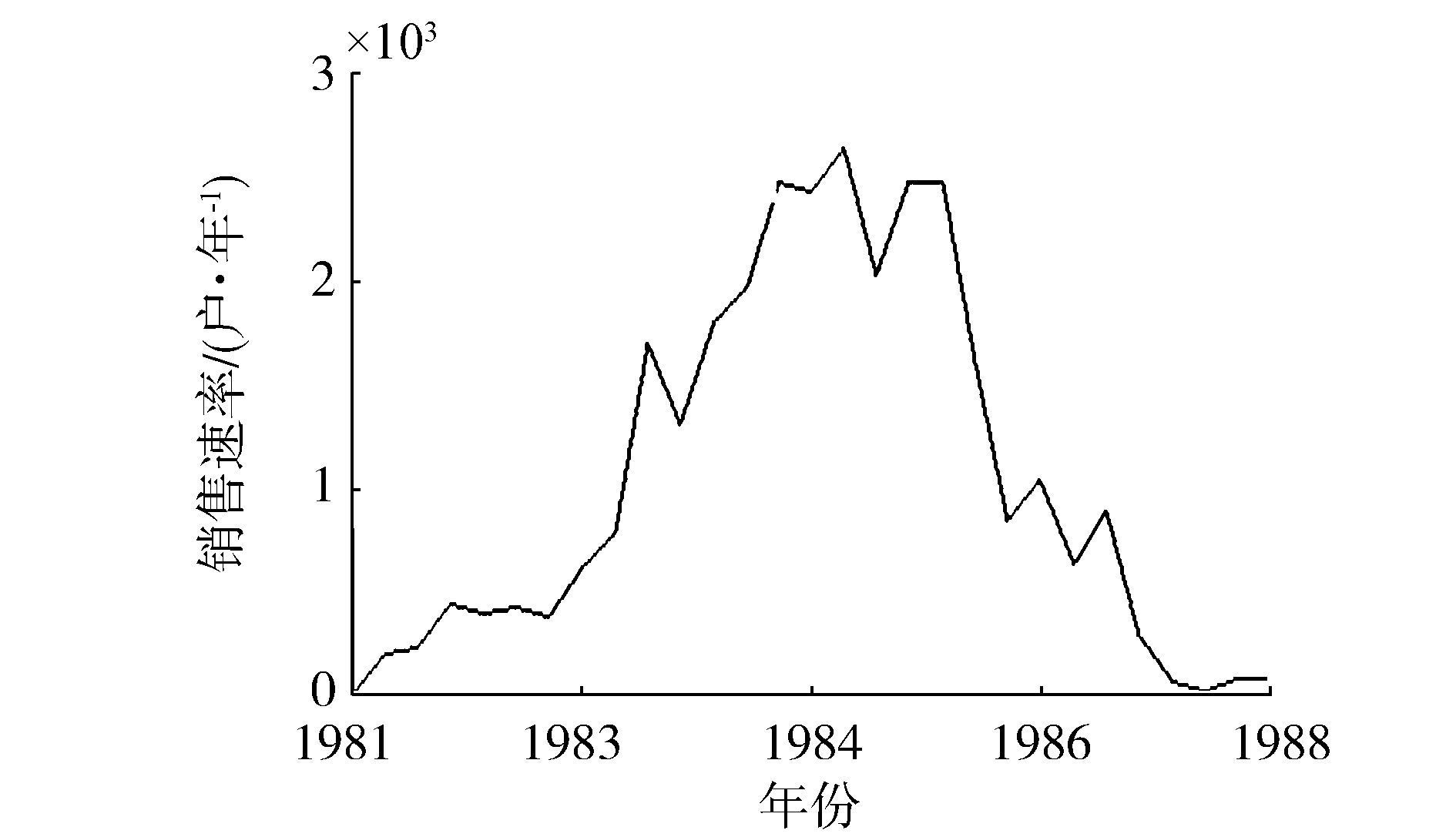图 15 历年销售速率 Fig.15 The selling rate of calendar year

2) 以SD的DYNAMO方程为依据，近似构造相关网内各物元特征的相关函数，并通过模拟，初步验证相关函数的可用性。

t+1时刻的潜在用户数量=t时刻的潜在用户数量-从t时刻到t+1时刻的时间间隔内产品的销售速率·时间间隔，即v3(t+1)=v3(t)-v4(tDT

t+1时刻的当前用户数量=t时刻的当前用户数量+从t时刻到t+1时刻的时间间隔内产品的销售速率·时间间隔，即v2(t+1)=v2(t)+v4(tDT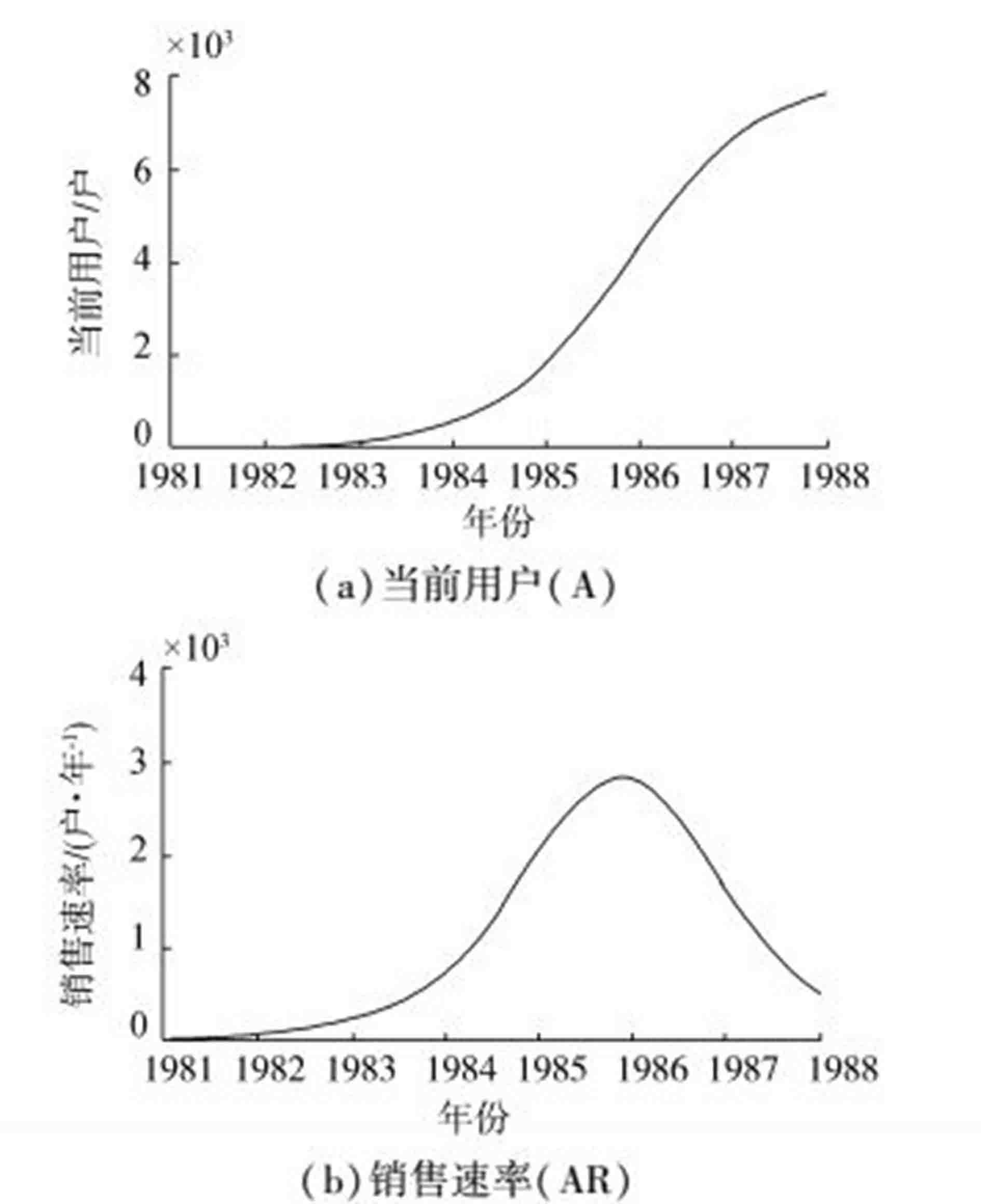图 16 模型运行结果 Fig.16 The results of the model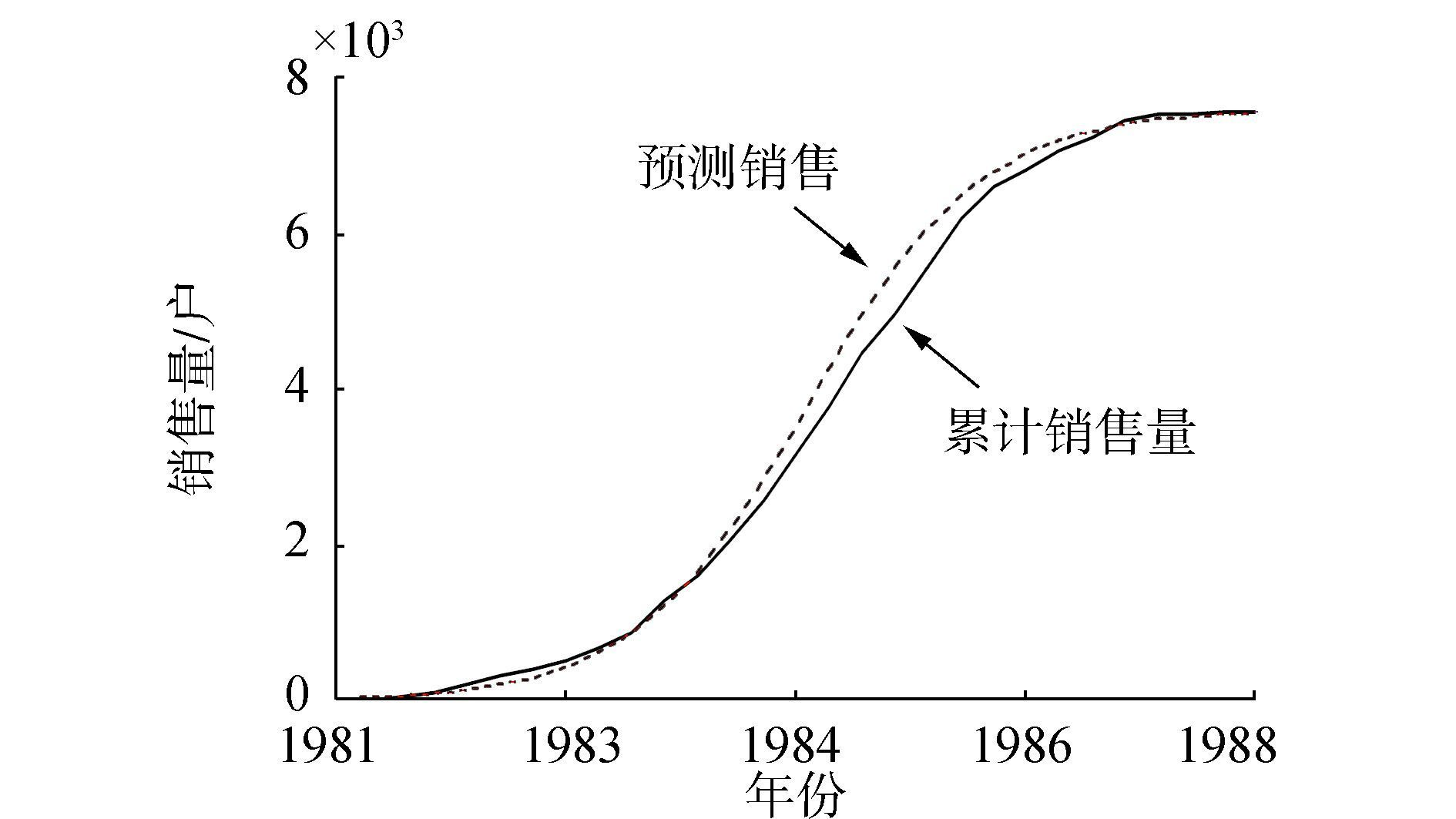图 17 销售量模拟对比 Fig.17 The comparison of modeling result of total sales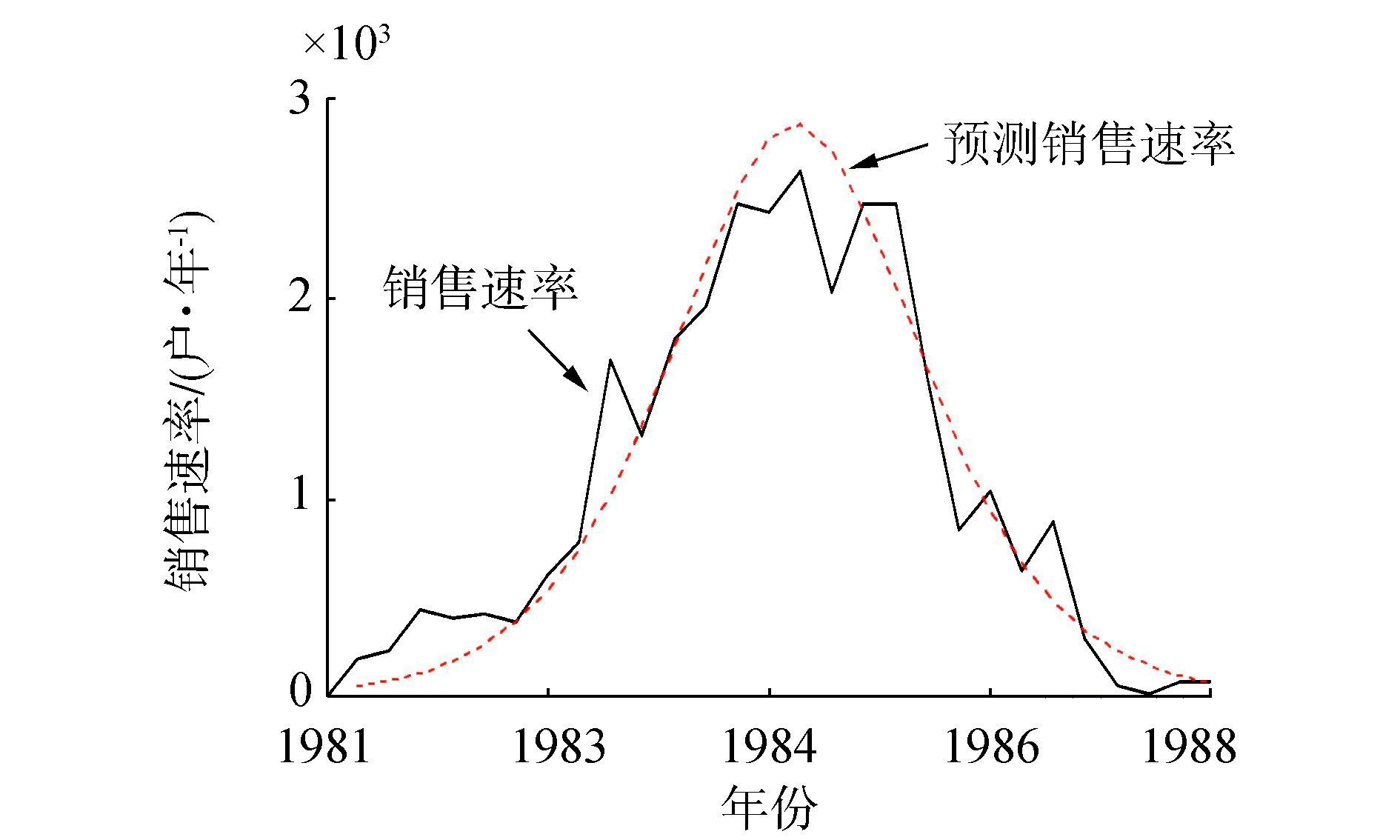图 18 销售速率模拟对比图 Fig.18 The comparison of modeling result of sales rate

 $\Re \left( t \right) \cdot f\left( {{v_5}\left( t \right)} \right) = \frac{1}{{{v_5}\left( t \right)}}$

 $\begin{array}{*{20}{c}} {{v_1}\left( t \right) = {v_2}\left( t \right) + {v_3}\left( t \right)}\\ {{v_3}\left( {t + 1} \right) = {v_3}\left( t \right) - {v_4}\left( t \right) \cdot {\rm{DT}}}\\ {{v_2}\left( {t + 1} \right) = {v_2}\left( t \right) + {v_4}\left( t \right) \cdot {\rm{DT}}}\\ {{v_4}\left( t \right) = \frac{{{v_5}\left( t \right) \cdot {v_2}\left( t \right) \cdot {v_3}\left( t \right)}}{{{v_1}\left( t \right)}}} \end{array}$
5 结论

1) 本文针对物元相关网，提出了相关网的系统动力学模型建模方法，为相关分析的实施提供了可智能化模拟分析的工具，在一定程度上为相关分析理论应用领域的拓展提供了可能。

2) 在此基础上提出了在动力学相关网模型中近似构造相关函数的方法。为相关函数未知情况下相关分析的实施提供了工具，在一定程度上弥补了相关分析理论运用中的局限性。

3) 以W公司新产品销售过程为例，通过对其建立相关网动力学模型，进行模拟分析以及近似构造未知相关函数，通过与对现实数据进行初步分析得出的信息对比，验证了其有效性。

  杨春燕, 蔡文. 可拓学[M]. 北京: 科学出版社, 2014: 43-133. (0)  汤龙, 杨春燕. 复杂基元相关网下的传导变换[J]. 智能系统学报, 2016, 11(1): 1-9. TANG Long, YANG Chunyan. Conductive transformation under complicated basic-element correlative network[J]. CAAI transactions on intelligent systems, 2016, 11(1): 1-9. (0)  杨春燕, 蔡文. 可拓学与矛盾问题智能化处理[J]. 科技导报, 2014, 32(36): 15-20. YANG Chunyan, CAI Wen. Extenics and intelligent processing of contradictory problems[J]. Science and technology review, 2014, 32(36): 15-20. DOI:10.3981/j.issn.1000-7857.2014.36.001 (0)  YANG Chunyan, CAI Wen. Extenics:theory, method and application[M]. Beijing: Science Press, 2013. (0)  蔡文, 杨春燕, 何斌. 可拓逻辑初步[M]. 北京: 科学出版社, 2004: 55-56. (0)  李立希, 杨春燕, 李铧汶. 可拓策略生成系统[M]. 北京: 科学出版社, 2006: 46-62. (0)  汪应洛. 系统工程[M]. 北京: 机械工业出版社, 2008: 86-102. (0)  张波, 虞朝晖, 孙强, 等. 系统动力学简介及其相关软件综述[J]. 环境与可持续发展, 2010(2): 1-4. ZHANG Bo, YUN Chaohui, SHUN Qiang, et al. Overview of system dynamics and related software[J]. Environment and sustanable development, 2010(2): 1-4. (0)  张力菠. 基于系统动力学的管理决策建模研究[D]. 南京: 南京理工大学, 2001: 8-19. ZHANG Libo. Research of management decision-making based on system dynamics modeling[D]. Nanjing:Nanjing University of Science and Engineering, 2001:8-19. http://cdmd.cnki.com.cn/Article/CDMD-10288-2002052955.htm (0)  陈国卫. 系统动力学应用研究综述[J]. 控制工程, 2012, 19(6): 921-928. CHEN Guowei. Application research overview of system dynamics[J]. Control engineering of China, 2012, 19(6): 921-928. (0)  毕克新, 孙德花, 李柏洲. 基于系统动力学的制造业企业产品创新与工艺创新互动关系仿真研究[J]. 创新管理, 2008(12): 75-80. BI Kexin, SUN Dehua, LI Baizhou. Product innovation and process innovation-a system dynamics-based simulation of the interaction in manufacturing enterprise[J]. Innovation management, 2008(12): 75-80. (0)  吴振华, 黎芸, 贵文龙, 等. 基于系统动力学的住宅价格预测研究[J]. 技术经济与管理研究, 2013(8): 101-105. WU Zhenghua, LI Yun, GUI Wenlong, et al. The forecast research of urban commodity residence price based on system dynamics[J]. Techno economics and management research, 2013(8): 101-105. (0)  郑士源. 基于系统动力学的两厂商投资微分博弈模拟[J]. 上海海事大学学报, 2006, 27(4): 70-74. ZHENG Shiyuan. Duopoly investment differential game simulation based on system dynamics[J]. Journal of Shanghai maritme university, 2006, 27(4): 70-74. (0)  梁吉业, 冯晨娇, 宋鹏. 大数据相关分析综述[J]. 计算机学报, 2016, 39(1): 1-18. LIANG Jiye, FENG Chenjiao, SONG Peng. A survey on correlation analysis of big data[J]. Chinese Journal of Computers, 2016, 39(1): 1-18. DOI:10.11897/SP.J.1016.2016.00001 (0)  夏幼明, 解敏, 周雯. 数据挖掘方法分析与评价[J]. 云南师范法学学报, 2003, 23(2): 7-16. XIA Youming, XIE Min, ZHOU Wen. Analysis and evaluation of data mining[J]. Journal of Yunnan normal university, 2003, 23(2): 7-16. (0)  郭秀娟. 数据挖掘方法综述[J]. 吉林建筑工程学院学报, 2004, 21(1): 49-53. GUO Xiujuan. Summary of data mining[J]. Journal of Jilin architectural and civil engineering, 2004, 21(1): 49-53. (0)  王惠忠, 彭安群. 数据挖掘研究现状及发展趋势[J]. 工矿自动化, 2011, 37(2): 29-32. WANG Huizhong, PENG Anqun. Study on status and development trends of data mining[J]. Industry and mine automation, 2011, 37(2): 29-32. (0)  胡文瑜, 孙志辉, 吴英杰. 数据挖掘取样方法研究[J]. 计算机研究与发展, 2011, 48(1): 45-54. HU Wenyu, SUN Zhihui, WU Yingjie. Study of sampling methods on data mining and stream mining[J]. Journal of computer research and development, 2011, 48(1): 45-54. (0)  崔广风. 数据挖掘中的统计方法及其应用研究[D]. 成都: 西南石油大学, 2014: 8-25. CUI Guangfeng. Study on statistical method and Its application in data mining[D]. Chengdu:Southwest Petroleum University, 2014:8-25. http://cdmd.cnki.com.cn/Article/CDMD-10615-1014411446.htm (0)  党辉. 基于系统动力学和云模型模糊数据挖掘的生态安全仿真预警[D]. 兰州: 西北师范大学, 2013: 29-49. DANG Hui. Ecological security simulation early warning based on system dynamics and fuzzy data mining on cloud model[D].Lanzhou:Northwest Normal University, 2013:29-49. http://cdmd.cnki.com.cn/Article/CDMD-10736-1014018998.htm (0)